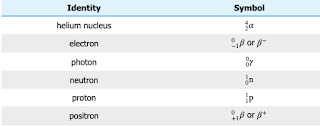## Monday, April 12, 2021

### Nuclear Chemistry Notation

General Chemistry Index

Where are we going with this? This page will assist in developing the ability to compare and contrast nuclear reactions with chemical reactions and to describe nuclear changes in matter, including fission, fusion, transmutations, and decays.

Nuclear Chemistry Notation

Okay, so, in nuclear chemistry, we are messing around inside the nucleus. Meaning we can change things up! Meaning not all of an element will have the same atomic mass! Isotopes and all that…

To show a more detailed picture of what is present within a particular atom, nuclear chemistry uses a more detailed means of notation.http://hyperphysics.phy-astr.gsu.edu/hbase/Nuclear/nucnot.html

There are three parts to the nuclear chemistry notation: The atomic symbol, the number of protons, and the total atomic mass.

And, it's hard to type! Sorry…

The notation consists of the atomic symbol written in "normal" size. At the top, left, is the total atomic mass much smaller. At the bottom left is the number of protons written much smaller.

I find it interesting that the number of protons dictates what the atomic symbol is. If the number of protons changes, the symbol changes, too. But, the number of protons and atomic mass have to be attached to something, so the atomic symbol makes sense.

I sort of think you could leave off the proton number, BUT!!!! when you start doing the reactions, it's really, really handy to have them noted already!

In the image below, you'll see a few examples.

Figure 1 is a uranium with 235 mass and 92 protons. (If it had a different number of protons, it wouldn't be uranium. This means it has 235 - 92 neutrons. Which is 143 neutrons.

Figure 2 is plutonium with a mass of 214 and 84 protons.They are sometimes typed like this:

23592U                21484Po

In future articles, the above typed format will be used.

_______________

There are also a couple of special symbols.

Those alpha particles

So, it's a He atom without electrons… It has a mass of 4 and has 2 protons. So… Yeah… Some sources will indicate them (in a way that can be typed) as…

He2+

…which makes sense. But, does not follow the normal notation system above, which is really, really handy when it come to the whole reaction thing.

So, there are two ways you'll often see the alpha particles notated for the purposes of nuclear reaction notation:

42α                42He

Both the 𝞪 and the He show the 4 and 2 for the mass and number of protons. However, with the He, it is not obvious that the electrons are missing. With the 𝞪 it is more certain.

Some sources will use the 𝞪 by itself without the mass/proton numbers. Now you know

_______________

Those beta particles

Recalling that we have two types of beta particles, we would expect two ways of notating them. Well…

Again, at a simple level, we can consider the negatively charged beta particle to be an electron. For the purposes of basic nuclear reaction concepts, this is acceptable. Thus, the ß- particle can be written the four ways shown in the image below:

e-       0-1 ß          ß-        0-1e

All of these notations represent an electron separated from an atom.

Looking at the second and fourth symbols above in light of the normal notation, it makes sense. The top number is atomic mass and the bottom number is how many protons. So, because it is an electron, it's a "negative proton" with a mass of zero. That makes sense, right?

The other beta particle (a positron) has a positive charge. Thus, the ß+ particle, can be written the two ways shown in the image below:

0+1 ß          ß+

In light of normal notation, then, it is a "massless" proton? Sort of? Just trying to connect ideas, here…

This particle is not seen often in introductory examples, but the notation is simple.

_______________

Protons and neutrons

No surprise here! Thankfully. The proton symbol is a "p" with a 1 at the top and bottom on the left, which means it has a mass of 1 and includes 1 proton. The neutron symbol is an "n" with a 1 at the top and a 0 at the bottom on the left.

Figure 1 below shows the notation for protons.
Figure 2 below shows the notation for a neutron.

11p                11n

_______________

Gamma rays

Some reactions will produce electromagnetic gamma ray radiation. The symbol for this is a greek letter, gamma:

γ

_______________

So what?Source
Nuclear chemistry notation is the vocabulary for nuclear reactions. So, when we start with some stuff and slam some more stuff into it, then stuff shoots out, we have a way of describing it.

Whereas each symbol sort of seems confusing seen alone, when put into a reaction, all the "up numbers" and "down numbers" will add up nicely.

It's actually a really, really handy notation system!